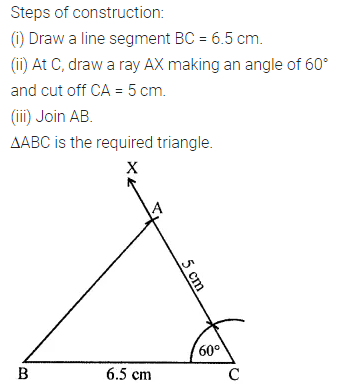ML Aggarwal Class 7 Solutions for ICSE Maths Model Question Paper 5 acts as the best resource during your learning and helps you score well in your exams.

## ML Aggarwal Class 7 ICSE Maths Model Question Paper 5

(Based on Chapters 13 to 16)
Time allowed: 1 Hour
Maximum Marks: 25
General Instructions

• Questions 1-2 carry 1 mark each.
• Questions 3-5 carry 2 marks each.
• Questions 6-8 cany 3 marks each
• Questions 9-10 carry 4 marks each.

Choose the correct answer from the given four options (1-2):
Question 1.
If the area of a triangle is 48 cm2 and the length of its base is 12 cm, then its height is
(a) 4 cm
(b) 8 cm
(c) 12 cm
(d) 16 cm
Solution: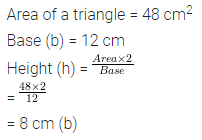Question 2.
The letter which has both line and rotational symmetry is
(a) W
(b) N
(c) X
(d) E
Solution:Question 3.
If the circumference of a circular sheet is 44 cm, then find its area.
Take π = $$\frac { 22 }{ 7 }$$.
Solution:Question 4.
Copy the given figure and draw all lines of symmetry.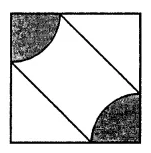Solution: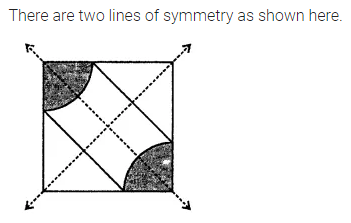Question 5.
The given figure shows a net of a die; the numbers inserted in each square indicate the number of dots on that face. Insert suitable numbers in the blank squares, remembering that the number of dots on opposite faces of a die should be 7.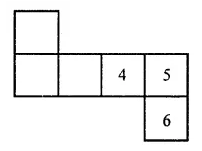Solution: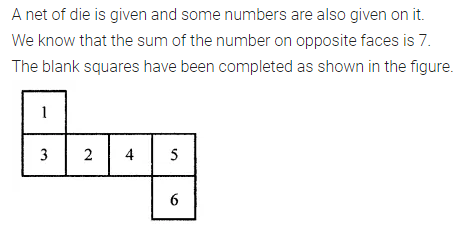Question 6.
A figure looks exactly the same at its original figure after a rotation of 72°. At what other angles will this figure appear the same? What can you say if the angle of rotation is (i) 60° (ii) 75°?
Solution: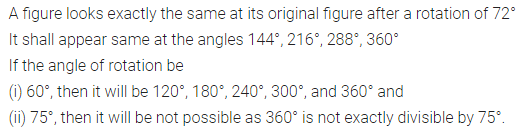Question 7.
In the given figure, a circle is inscribed in a semicircle of radius 7 cm. Find the area of the region.
Take π = $$\frac { 22 }{ 7 }$$.Solution: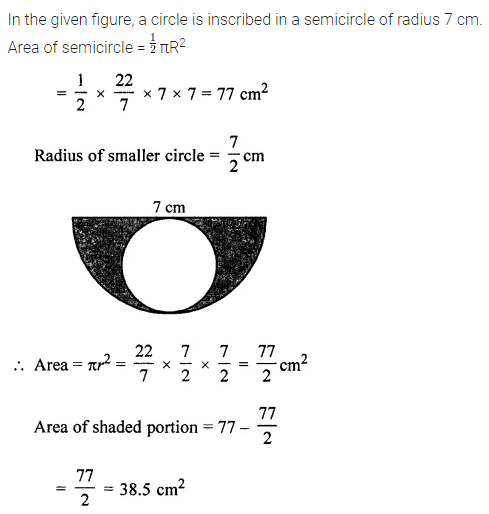Question 8.
Draw an isometric sketch of a cuboid of length 5 units, breadth 4 units and height 3 units.
Solution:Question 9.
In the given figure, ABCD is a parallelogram with AB = 6 cm and AD = 5 cm. If DM is perpendicular to AB and the area of parallelogram ABCD is 24 cm2, find the length of AM.Solution: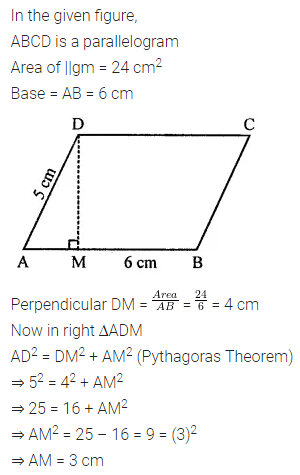Question 10.
By using ruler and compass, construct a triangle ABC with BC = 6.5 cm, AC = 5 cm and ∠C = 60°.
Solution: